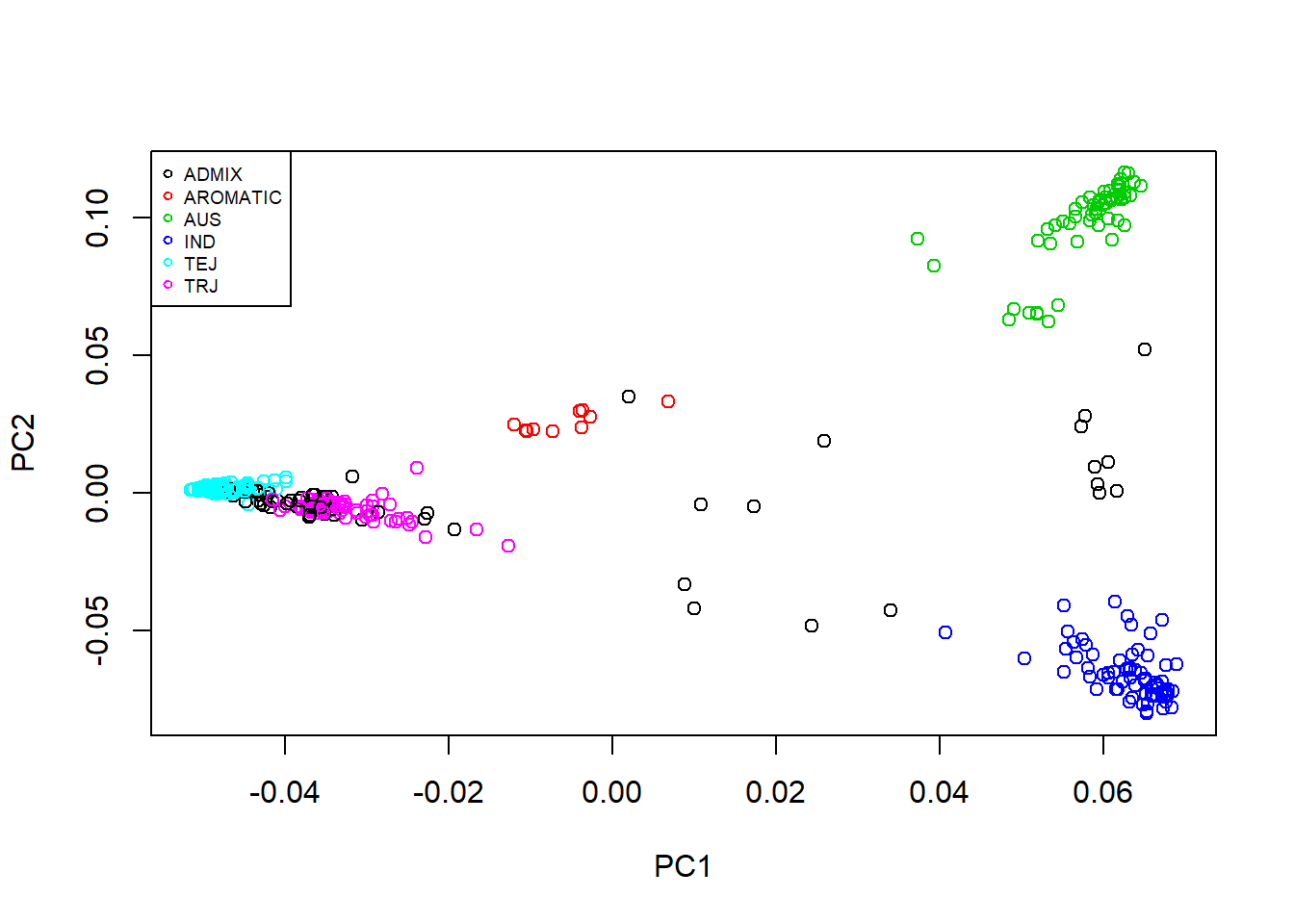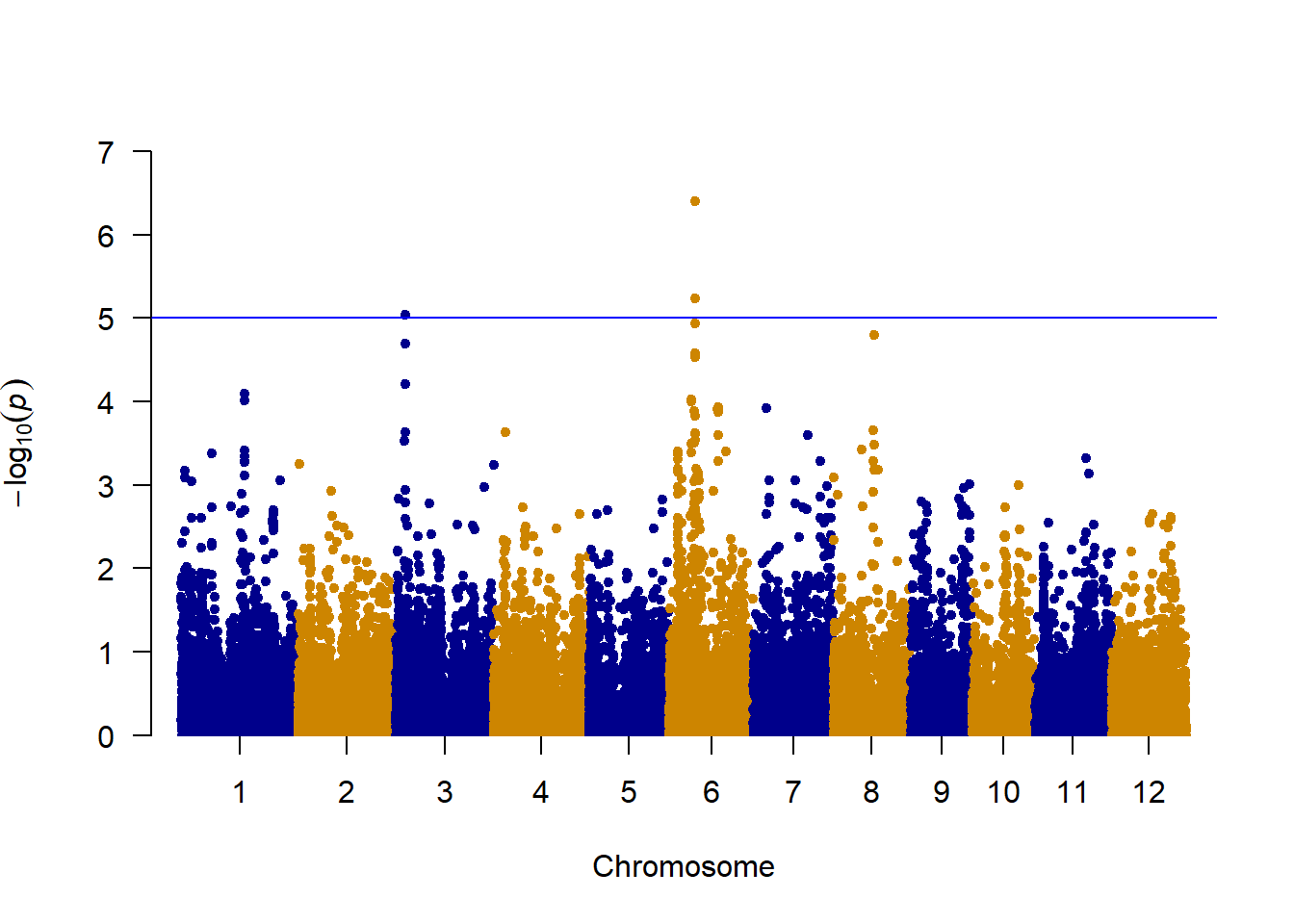## R Markdown

    library(BGLR)
p=out$p n=out$n
out=out$x Recode snp to 0,1,2 format using allele out[out==2]=NA out[out==3]=2 W <- matrix(out, nrow=p, ncol=n, byrow=TRUE) W <- t(W) dim(W) ##  413 36901 Accession IDs The accession IDs (individual IDs) are included in .fam file. fam <-read.table("C:/Users/wasim/Desktop/Lectures/Practical/RiceDiversity.44K.MSU6.Genotypes_PLINK/RiceDiversity_44K_Genotypes_PLINK/sativas413.fam") head(fam) ## V1 V2 V3 V4 V5 V6 ## 1 081215-A05 1 0 0 -9 -9 ## 2 081215-A06 3 0 0 -9 -9 ## 3 081215-A07 4 0 0 -9 -9 ## 4 081215-A08 5 0 0 -9 -9 ## 5 090414-A09 6 0 0 -9 -9 ## 6 090414-A10 7 0 0 -9 -9  rownames(W) <- fam$V2

Phenotype data We will use the read.table function to read the phenotype file RiceDiversity_44K_Phenotypes_34traits_PLINK.txt from here. phenotypes

rice.pheno <- read.table("http://www.ricediversity.org/data/sets/44kgwas/RiceDiversity_44K_Phenotypes_34traits_PLINK.txt", header=TRUE, stringsAsFactors = FALSE, sep = "\t")
table(rownames(W) == rice.pheno$NSFTVID) ## ## TRUE ## 413  y <- matrix(rice.pheno$Flowering.time.at.Arkansas) # # use the first trait
rownames(y) <- rice.pheno$NSFTVID index <- !is.na(y) y <- y[index, 1, drop=FALSE] # 374 W <- W[index, ] # 374 x 36901 table(rownames(W) == rownames(y)) ## ## TRUE ## 374 Population structure gp <-read.csv("http://ricediversity.org/data/sets/44kgwas/RiceDiversity.44K.germplasm.csv", header = TRUE, skip = 1, stringsAsFactors = FALSE) # 431 x 12 gp2 <- gp[match(rownames(y), gp$NSFTV.ID), ]
table(rownames(y) == gp2$NSFTV.ID) ## ## TRUE ## 374  plot(gp2$PC1, gp2$PC2, xlab="PC1", ylab="PC2", col=c(1:6)[factor(gp2$Sub.population)])
legend(x="topleft", legend = levels(factor(gp2\$Sub.population)), col=c(1:6), pch=1, cex=0.6)Quality control Genotype imputation Replace missing marker genotypes with mean values. Then store the marker genotypes in a matrix object W.

for (j in 1:ncol(W)){
W[,j] <- ifelse(is.na(W[,j]), mean(W[,j], na.rm=TRUE), W[,j])
}
sum(W[,1]) # sum of A allele in the first SNP
##  224.6005
    sum(W[,2]) # sum of A allele in the second SNP
##  226.6059
    p <- colSums(W) / (2 * nrow(W)) # or colMean(X) / 2

Minor allele frequency (MAF) Perform a quality control analysis by removing markers with MAF < 0.05. How many markers are removed? Save the filtered genotype matrix in W2.

    maf <- ifelse(p > 0.5, 1-p, p)
maf.index <- which(maf < 0.05)
W2 <- W[, -maf.index]  # 374 x 33558 

Genome-wide association studies Fit a single-marker-based linear mixed model by using the GWAS function in the rrBLUP R package. Report the -log10 of p-values for SNP effects. install.packages(“rrBLUP”)

library(rrBLUP)
## Warning: package 'rrBLUP' was built under R version 3.3.3
    map <- read.table("C:/Users/wasim/Desktop/Lectures/Practical/RiceDiversity.44K.MSU6.Genotypes_PLINK/RiceDiversity_44K_Genotypes_PLINK/sativas413.map", header = FALSE, stringsAsFactors = FALSE)
my.geno <- data.frame(marker=map[,2], chrom=map[,1], pos=map[,4], t(W-1), check.names = FALSE) # W = \in{-1, 0, 1}
my.pheno <- data.frame(NSFTV_ID=rownames(y), y=y)

rel <- GWAS(my.pheno, my.geno, min.MAF=0.05, P3D=TRUE, plot=FALSE)
##  "GWAS for trait: y"
##  "Variance components estimated. Testing markers."

Manhattan plot install.packages(“qqman”)

library(qqman)
manhattan(x = rel, chr = "chrom", bp = "pos", p = "y", snp = "marker", col = c("blue4", "orange3"), logp = FALSE)END